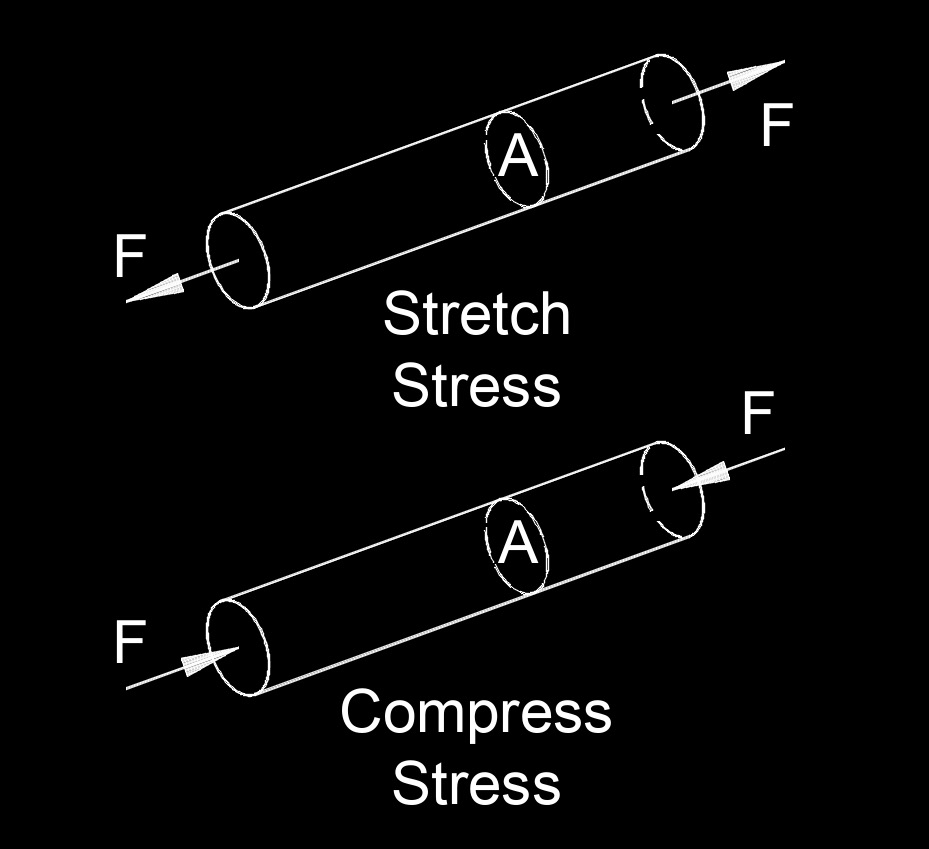# Stress

Written by Jerry Ratzlaff on . Posted in Classical MechanicsStress, abbreviated as $$\sigma$$ (Greek symbol sigma), is the force per unit area of cross-section.  The maximum stress of a material before it breaks is called breaking stress or ultimate tensial stress.

### Stress formula

$$\large{ \sigma = \frac{F}{A_c} }$$

$$\large{ \sigma = \lambda \; \epsilon }$$     (elastic modulus)

$$\large{ \sigma = E \; \epsilon }$$     (Young's modulus)

Where:

$$\large{ \sigma }$$  (Greek symbol sigma) = stress

$$\large{ A_c }$$ = area cross-section

$$\large{ \lambda }$$  (Greek symbol lambda) = elastic modulus

$$\large{ F }$$ = force

$$\large{ \epsilon }$$  (Greek symbol epsilon) = strain

$$\large{ E }$$ = Young's modulus